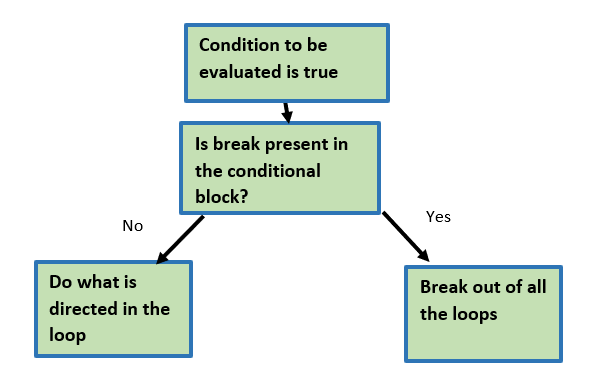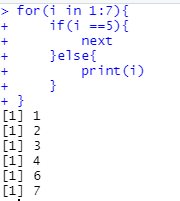Search
•Ekta Aggarwal

# Loops in R

Updated: Jan 13, 2021

There are two types of loops in R:

• for loop

• while loop

In this tutorial we shall be covering both of them in detail.

## For loop

Syntax 1: In this syntax, i is the iterator variable which takes values from 1: N, you have to specify N - which must be an integer.

for(i in 1:N){ <Do Something> }

### Example: Following for loop prints the value of i (i.e. from 1 to 10)

```for(i in 1:10){
print(i)
}```Note: When you are working in loops and if else then you need to explicity specify print( ) command. In the example given below, no output would be printed.

```for(i in 1:10){
i
}```

### Example: We create a vector named 'my_vector'. Our for loop prints the ith element of the vector.

Note the iterator variable i varies till length of my_vector which is 3.

```my_vector = c("Urban","Semi-Urban","Rural","Hilly")

for(i in 1:length(my_vector)){
print(my_vector[i])
}```Syntax 2: In this syntax, i is the iterator variable which takes values equal to my_vector (not 1 to 3)

```for(i in my_vector){
print(i)
}```

### Example: Creating new variables in a dataframe using for loops

Use airquality dataset and convert temerature (in Fahrenheit) to Celcius by creating a new column temp_in_celc.

In the example below, our iterator 'i' varies till nrow(airquality).

Note: While creating new variables using for loops we have to define [i] th element ,

our loop firstly takes the value i=1 and then updates first value in vector temp_in_celc using Temp , then the value of i gets incremented to 2 (i=2) and temp_in_celc gets calculated using Temp and so on.

```View(datasets::airquality)

for(i in 1:nrow(airquality)){
airquality\$temp_in_celc[i] = (airquality\$Temp[i] -32) *5/9
}```

### Example: Creating a new variable using for loops

Note: When you are creating a new variable then following will result in Error

```for(i in 1:nrow(airquality)){
temp_in_celc[i] = (airquality\$Temp[i] -32) *5/9
}```

While creating a new object (variable) we have to firstly specify it as NULL and then need to use the for loop.

```temp_in_celc = NULL

for(i in 1:nrow(airquality)){
temp_in_celc[i] = (airquality\$Temp[i] -32) *5/9
}```

### Nested For loops

A for loop inside a for loop is called a nested for loop.

In the illustration given below here 'i' takes the value 1 and 'j' takes values as 1 to 3. Then for i=2, j iterates over 1 to 4 and so on.

```for (i in 1:4) {
for (j in 1:3) {
print(paste("i=", i, "; j=", j))
}
}```

### Example:

Create a new variable Description, which takes values as:

if Day<= 15, then First half of the month;

otherwise Second half of the month

```for(i in 1:nrow(airquality)){
if(airquality\$Day[i] <= 15){
airquality\$Description[i] = "First half of month"
}else{
airquality\$Description[i] = "Second half of month"
}
}```

## While loop

Using while loops, one needs to define the iterator seperately outside the loop and the value of iterator needs to be incremented specifically inside the loop. While loop is an entry control loop - which means it checks a condition first, if the condition gets satisfied then only the loop will be executed.

```i = 1

while(i<= nrow(airquality))
{
if(airquality\$Day[i] <= 15){
airquality\$Description2[i] = "First half of month"
} else {
airquality\$Description2[i] = "Second half of month"
}
i=i+1
}```

Note: Every for loop can be written as while loop, but the converse might not always be feasible.

## Break statement

While working with loops we might need to terminate all the loops if we encouncter one single condition, in such a scenario break statement is of great help.In the following example, as soon as the i takes value 5, all of our loops get terminated.

```for(i in 1:7){
if(i ==5){
break
}else{
print(i)
}
}```## Next statement

Suppose we want to skip the iterator of an iterator if we encouncter one single condition, in such a scenario NEXT statement is of great help.In the following example, as soon as the i takes value 5, out i=5 iteration gets skipped and then i takes the value as 6.

```for(i in 1:7){
if(i ==5){
next
}else{
print(i)
}
}```Tags:

See All